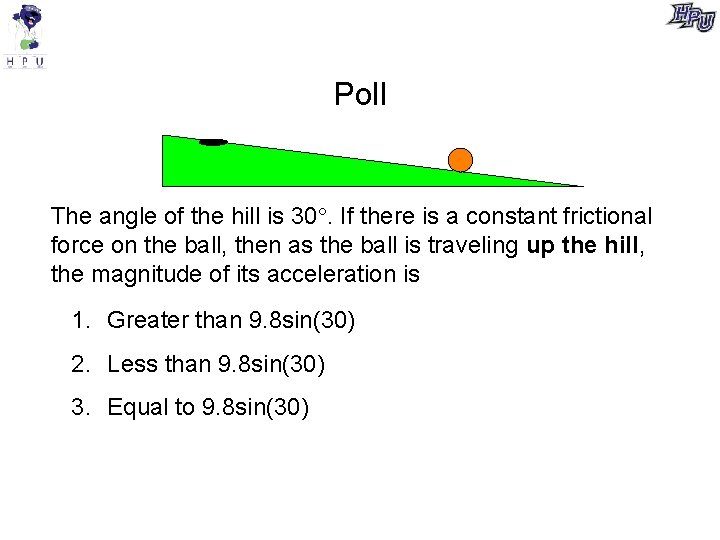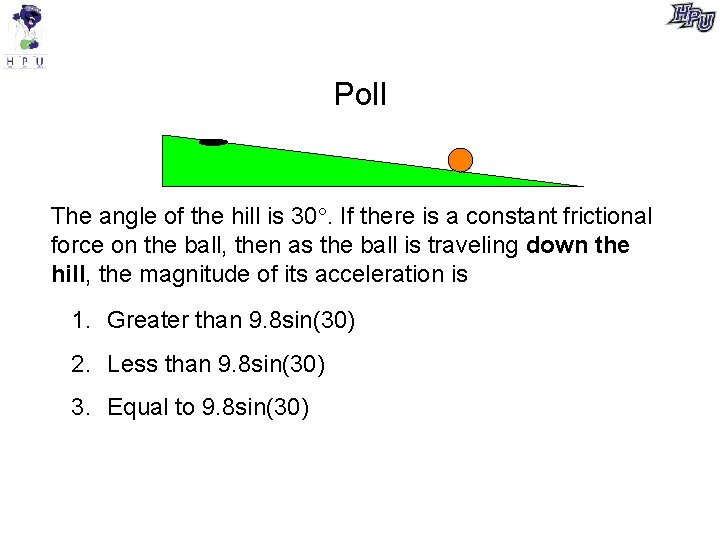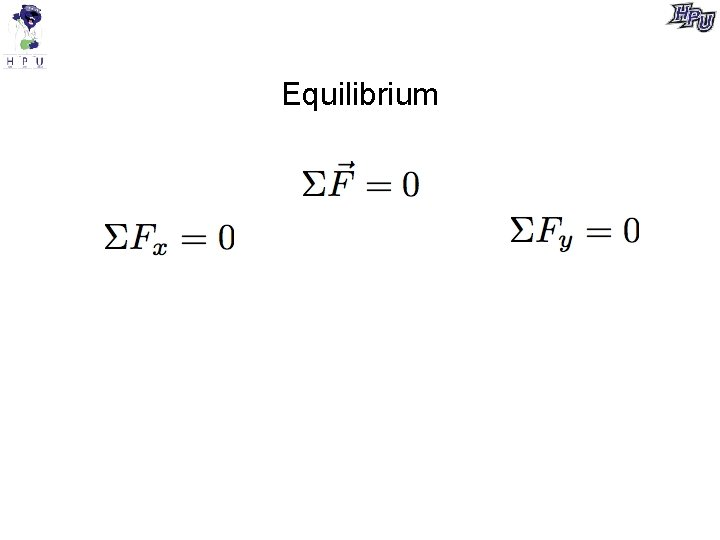# Poll The angle of the hill is 30

• Slides: 12Poll The angle of the hill is 30. If the ball is moving up the hill, what is its acceleration, if the +x direction is defined to be “to the right, down the hill”? 1. 9. 8 sin(30) 2. 9. 8 cos(30) 3. -9. 8 sin(30) 4. -9. 8 cos(30) 5. None of the abovePoll The angle of the hill is 30. If the ball is moving down the hill, what is its acceleration, if the +x direction is defined to be “to the right, down the hill”? 1. 9. 8 sin(30) 2. 9. 8 cos(30) 3. -9. 8 sin(30) 4. -9. 8 cos(30) 5. None of the aboveMotion on a hill with friction v Sketch a force diagram if the ball is moving up the hill v Sketch a force diagram if the ball is moving down the hillMotion on a hill with friction v What is its acceleration when moving up the hill? v What is its acceleration when moving down the hill?Poll The angle of the hill is 30. If there is a constant frictional force on the ball, then as the ball is traveling up the hill, the magnitude of its acceleration is 1. Greater than 9. 8 sin(30) 2. Less than 9. 8 sin(30) 3. Equal to 9. 8 sin(30)Poll The angle of the hill is 30. If there is a constant frictional force on the ball, then as the ball is traveling down the hill, the magnitude of its acceleration is 1. Greater than 9. 8 sin(30) 2. Less than 9. 8 sin(30) 3. Equal to 9. 8 sin(30)EquilibriumStanding on a hill If you stand vertically on a bathroom scale on a hill that is inclined at an angle of 30 , what will the scale read? What is the frictional force on you?Rings Suppose that each of these two rings each make an angle of 10 (outward from the vertical). What is tension in the rings if his mass is 70 kg?Friction FN, on book by table Ff The tangential (i. e. parallel) component of the force on the book by the table is friction. Friction depends on: (1) the surfaces in contact (2) the normal component of the contact forceCoefficient of friction If the surfaces are sliding past each other If surfaces are not sliding past each other but are just about to slide If surfaces are not sliding and are not about to slideExample